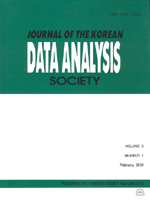상세검색
최근 검색어 전체 삭제
다국어입력
즐겨찾기0KCI등재 학술저널

# On the Estimation of the Distribution Function Using Extreme Median Ranked Set Sampling

• 등재여부 : KCI등재
• 2005.04
• 429 - 439 (11 pages)

Regarding Simple Random Sample (SRS), the relationship between Extreme Ranked Set Samples (ERSSs) and Median Ranked Set Sample (MRSS) was studied in detail by Samawi and AL-Sagheer(2001). What we consider in this paper is the Extreme Median Ranked Set Sample (EMRSS) of SRS and Ranked Set Sampling (RSS), which is a mixed form of ERSSs and MRSS. We prove in this paper that the distribution function estimator of EMRSS for a random variable X is more efficient than SRS and RSS estimator for a given x. The Relative Precision (RP) of EMRSS, SRS, and RSS was calculated respectively.

1. Introduction

2. Extreme Median Ranked Set Sampling

3. Estimation of Distribution Function

4. Conclusions and Further Works

References